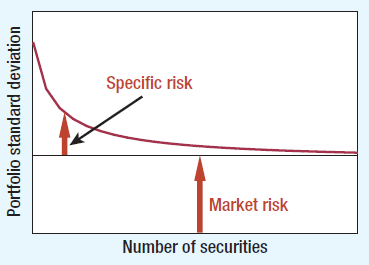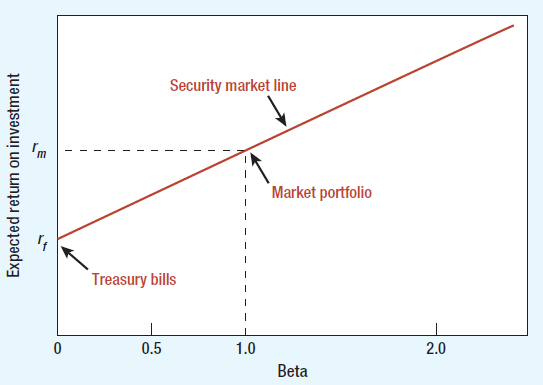# CAPM Calculator (Capital Asset Pricing Model)

Created by Tibor Pal, PhD candidate
Reviewed by Bogna Szyk
Based on research by
Brealey, R.; Myers, S.; Allen, F. Principles of Corporate Finance. Mc Graw Hill Education (2016)
Last updated: Jul 20, 2022

This Capital Asset Pricing Model calculator or CAPM formula helps you to find out what is the expected return of your asset or investment according to its inherent risk level.

If you already know how to calculate CAPM, you may have a look at WACC which helps you to calculate a firm's cost of capital with also taking into account the debt dimension of an investment.

## What is CAPM?

The CAPM model (Capital Asset Pricing Model) is one of the foundational models in finance designed to specify the appropriate required rate of return of a financial asset or investment.

Considering that CAPM is mostly applied to financial assets (securities), in the following, we will focus on these type of assets.

Since the core of the CAPM model is the "beta" parameter, which is the most widely employed financial measure used by academic researchers and practitioners, we shall get a bit more insight in its function.

The starting point is that the CAPM model is based on the perception that the total risk of a particular asset can be separated into two distinct parts:

• Market risk, undiversifiable market risk or systematic risk, assigned by “beta” measures the variation of asset value in response to a change in the aggregated value of all assets in the economy.

• Specific risk or diversifiable risk, which can be attributed to the peculiarity of an asset and its close environment.

The below graph represents the alteration of these risks depending on the number of assets in the portfolio.Source: Brealey, R., Myers, S., Allen, F. (2016). Principles of Corporate Finance. Mc Graw Hill Education

On the graph you can see that the specific risk, unlike the market risk, can be reduced by increasing the number of assets included in the portfolio; more precisely, this type of risk can be eliminated by diversification. Therefore, according to the CAPM model, the required rate of return should depend on its market risk or “beta”: the higher systematic risk should imply a higher expected return for the asset.

Wise investors don't take risks just for fun, right? Since they play with real money, they require a higher return from the market portfolio than from a risk-free asset. More specifically, according to the CAPM, the required rate of return equals the risk-free interest rate plus a risk premium that depends on beta and the market risk premium.

These relations can be illustrated with the CAPM formula:

• risk premium = beta * (market risk premium)

• market risk premium = Rm - Rf

To get a better insight, the below graph displays the visual representation of the CAPM equation.Source: Brealey, R., Myers, S., Allen, F. (2016). Principles of Corporate Finance. Mc Graw Hill Education

You can see on the graph that the intercept of the beta of 1 and the market return represents the market portfolio. It means that a well-diversified portfolio with such asset moves together with the market.

A beta of 0.5 has below average market risk, which means that a well-diversified portfolio of these assets tends to be half as sensitive to market changes.

Since the expected risk premium on each investment is proportional to its beta, each investment should lie on the sloping security market line which connects the risk-free return (treasury bills on the graph) and the market portfolio.

## CAPM formula

If you want to calculate the CAPM for your asset or investment, you need to use the following CAPM formula:

R = Rf + risk premium

risk premium = beta * (Rm - Rf)

where:

• R stands for the expected rate of return of an asset or investment,
• Rf is the risk-free interest rate, typically taken as the yield on a long-term government bond in the country where the project is based,
• Rm is the broad market return, and
• Beta is the parameter of the market risk.

## CAPM model in practice

To demonstrate the mechanism of the CAPM model more vividly, it might be worth to look into a real-world example.

Let's say, you are a shareholder who is considering to invest in a particular stock to widen your portfolio and you would like to evaluate the effect of the new share on your portfolio.

Let's recall the CAPM formula.

R = Rf + beta * (Rm - Rf)

Since Treasury bills are typically considered as a risk-free investment, we can use its yield for the calculation, thus Rf = 2.4% for the 3-month US Treasury Bill.

Because calculation behind the parameter beta involves more in-depth research with some statistics, we will rely on a published average value of beta of a well-known company, Walmart Inc. Therefore, beta = 0.47, which means that the sensitivity of Walmart share's return for changes in the aggregate value of assets share is around half as the average market.

The last variable which we need for the calculation is the market return. In case of stocks, to estimate the market return we can use the long-term average return of S&P 500 or the Dow Jones, which can be considered as an index to reflect the overall return characteristic of the stock exchange as a whole. For the S&P 500 since its initiation in 1928 through 2017 is approximately 10%, so Rm = 10%.

Substituting the values into the CAPM formula, we attain the following:

R = 2.4 + 0.47 * (10 - 2.4) = 5.97

Notice that the market risk premium is 7.6% and the risk premium of a Walmart share is 3.57%.

Thus, with rounding, R = 6%, which means that an expected return of a Walmart share is around 6%.

Recognize also that if we assume that the beta of your initial portfolio was more then 0.47, widening your portfolio by Walmart share lowers the beta of the new portfolio. In other words, it reduces the reactiveness of the new portfolio's return to aggregate changes in the economy.

## Interesting facts and limitations

• William Sharpe, who designed the Sharpe ratio also, was awarded the Nobel Prize in Economics in 1990 for the development of the Capital Asset Pricing Model (CAPM). In 2002, this prize was given to Daniel Kahneman for developing the Prospect Theory (PT), which contradicts Expected Utility Theory (EUT) on which the CAPM is based.

• Since the CAPM model strictly relies on assumptions about investor behaviors, risk and return distribution, and market fundamentals which often depart from the reality, CAPM is heavily criticized both theoretically and empirically. Still, understanding the concept behind the CAPM model can help you to perceive the relationship between risk and return and to become a smarter investor.

Tibor Pal, PhD candidate
Risk-free interest rate (Rf)
%
Broad market return (Rm)
%
Beta
Risk premium of the asset
%
Expected rate of return (R)
%
People also viewed…

Addiction calculator tells you how much shorter your life would be if you were addicted to alcohol, cigarettes, cocaine, methamphetamine, methadone, or heroin.

### Chilled drink

With the chilled drink calculator you can quickly check how long you need to keep your drink in the fridge or another cold place to have it at its optimal temperature. You can follow how the temperature changes with time with our interactive graph.

### Cross price elasticity

Cross price elasticity calculator shows you what the correlation between the price of product A and the demand for product B is.

### Variable annuity

Use the variable annuity calculator to estimate a subject of your interest in a variable annuity construction.